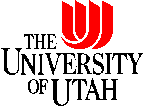Department of Mathematics, University of UtahDepartment of Mathematics, College of Science, University of Utah, Salt Lake City, Utah 84112-0090, USA. 801-581-6851, 801-581-4148 (fax)## 84112

Our zip code, 84112, has the prime factorization
84112 = 2 4 *7*751.

There are 8198 prime numbers less than 84112. The number 84112 can be written in 620 ways as the sum of two prime numbers.For example,

84112 = 42023 + 42089.

The next smallest prime number is 84089, and the next largest 84121.

You can find out these things right here on line using the prime machine.

## 84112-0090

The situation is a little more complicated for our complete 9 digit zip code 84112-0090, where this number is interpreted as

n=841,120,090

On all machines I know of the prime machine runs out of memory when attempting to disect that number. However, this FORTRAN code will compute such data for large numbers (usually up to 2,147,483,647 on a 32 bit system) in a very short time.

It turns out that there are 43,149,915 primes less than n. n can be written as the sum of two odd primes in 1,945,268 different ways. For example,

n = 420,559,259+ 420,560,831.

The prime twin closest to but below n is 841,119,899 and 841,119,901, and there is a total of 2,931,590 prime twins below n.

The prime factorization of n is
n = 2 * 5 * 84,112,009

University of Utah, Department of Mathematics, 155 S 1400 E Rm 233, Salt Lake City, UT 84112-0090, USA 801-581-6851, 801-581-4148 (fax)       [15-Jan-1998].